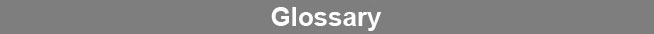Select Page### Poisson Distribution

A poisson distribution is a distribution of random occurrences in which one occurrence has no influence on any other occurrence. The variance of a poisson distribution is equal to its mean and therefore the standard deviation is equal to the square root of the mean of the distribution. Radioactive decay measurements follow a poisson distribution and therefore have a lower measurement error when more counts are accumulated.www.brendan.com